# Bash Range: How to iterate over sequences generated on the shell

You can iterate the sequence of numbers in bash by two ways. One is by using seq command and another is by specifying range in for loop. In seq command, the sequence starts from one, the number increments by one in each step and print each number in each line up to the upper limit by default. If the number starts from upper limit then it decrements by one in each step. Normally, all numbers are interpreted as floating point but if the sequence starts from integer then the list of decimal integers will print. If seq command can execute successfully then it returns 0, otherwise it returns any non-zero number. You can also iterate the sequence of numbers using for loop with range. Both seq command and for loop with range are shown in this tutorial by using examples.

## The options of seq command:

You can use seq command by using the following options.

-w

This option is used to pad the numbers with leading zeros to print all numbers with equal width.

-f format

This option is used to print number with particular format. Floating number can be formatted by using %f, %g and %e as conversion characters. %g is used as default.

-s string

This option is used to separate the numbers with string. The default value is newline (‘\n’).

### Examples of seq command:

You can apply seq command by three ways. You can use only upper limit or upper and lower limit or upper and lower limit with increment or decrement value of each step . Different uses of the seq command with options are shown in the following examples.

#### Example-1: seq command without option

When only upper limit is used then the number will start from 1 and increment by one in each step. The following command will print the number from 1 to 4.

\$ seq 4

Output: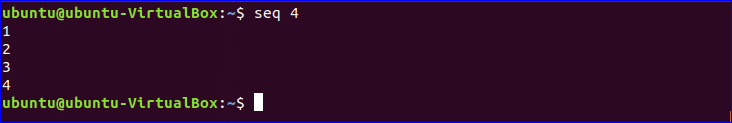When the two values are used with seq command then first value will be used as starting number and second value will be used as ending number. The following command will print the number from 7 to 15.

\$ seq 7 15

Output: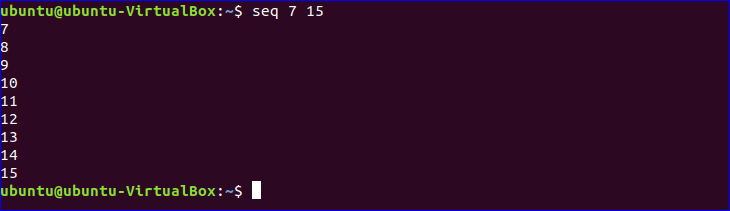When you will use three values with seq command then the second value will be used as increment or decrement value for each step. For the following command, the starting number is 10, ending number is 1 and each step will be counted by decrementing 2.

\$ seq 10 -2 1

Output: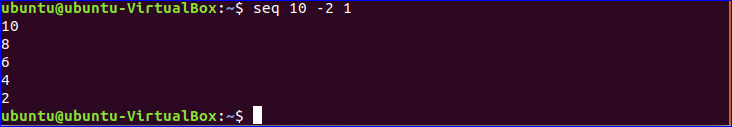#### Example-2: seq with –w option

The following command will print the output by adding leading zero for the number from 1 to 9.

\$ seq -w 0110

Output: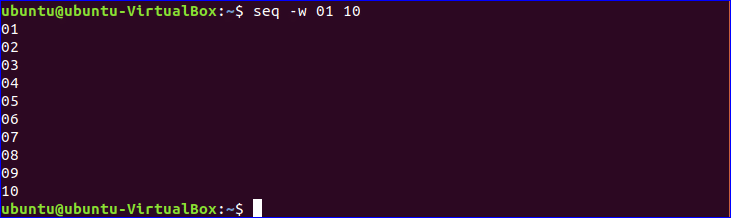#### Example-3: seq with –s option

The following command uses “-“ as separator for each sequence number. The sequence of numbers will print by adding “-“ as separator.

\$ seq -s - 8

Output: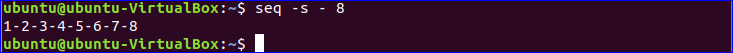#### Example-4: seq with -f option

The following command will print 10 date values starting from 1. Here, “%g” option is used to add sequence number with other string value.

\$ seq -f "%g/04/2018" 10

output: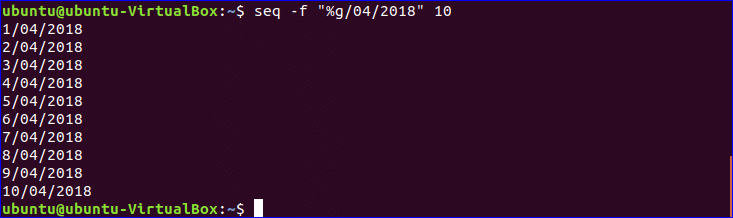The following command is used to generate the sequence of floating point number using “%f” . Here, the number will start from 3 and increment by 0.8 in each step and the last number will be less than or equal to 6.

\$ seq -f "%f" 3 0.8 6

Output: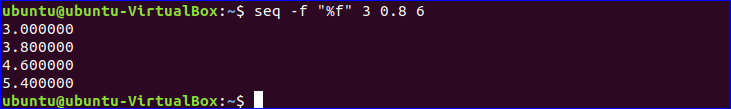#### Example-5: Write the sequence in a file

If you want to save the sequence of number into a file without printing in the console then you can use the following commands. The first command will print the numbers to a file named “seq.txt”. The number will generate from 5 to 20 and increment by 10 in each step. The second command is used to view the content of “seq.txt” file.

\$ seq 5 10 20 | cat &gt; seq.txt
\$ cat seq.txt

Output: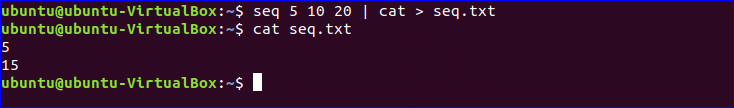### Example-6: Using seq in for loop

Suppose, you want to create files named fn1 to fn10 using for loop with seq. Create a file named “sq1.bash” and add the following code. For loop will iterate for 10 times using seq command and create 10 files in the sequence fn1, fn2,fn3…..fn10.

#!/bin/bash
for i in `seq 10`
do
touch fn.\$i
done

Output:

Run the following commands to execute the code of the bash file and check the files are created or not.

\$ bash sq1.bash
\$ ls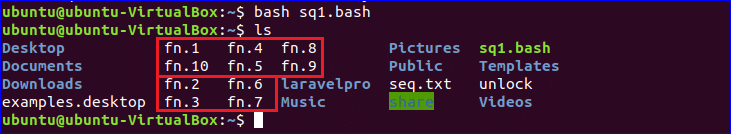### Examples of for loop with range:

#### Example-7: For loop with range

The alternative of seq command is range. You can use range in for loop to generate sequence of numbers like seq. Write the following code in a bash file named “sq2.bash”. The loop will iterate for 5 times and print the square root of each number in each step.

#!/bin/bash
for n in {1..5}
do
((result=n*n))
echo \$n square=\$result
done

Output:

Run the command to execute the script of the file.

\$ bash sq2.bash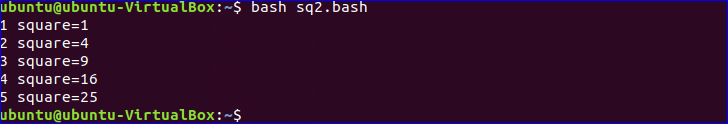### Example-8: For loop with range and increment value

By default, the number is increment by one in each step in range like seq. You can also change the increment value in range. Write the following code in a bash file named “sq3.bash”.  The for loop in the script will iterate for 5 times, each step is incremented by 2 and print all odd numbers between 1 to 10.

#!/bin/bash
echo "all odd numbers from 1 to 10 are"
for i in {1..10..2}
do
echo \$i;
done

Output:

Run the command to execute the script of the file.

\$ bash sq3.bash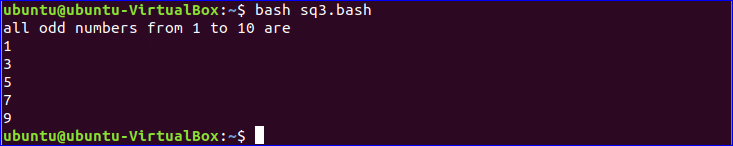If you want to work with the sequence of numbers then you can use any of the options that are shown in this tutorial. After completing this tutorial, you will be able to use seq command and for loop with range more efficiently in your bash script.